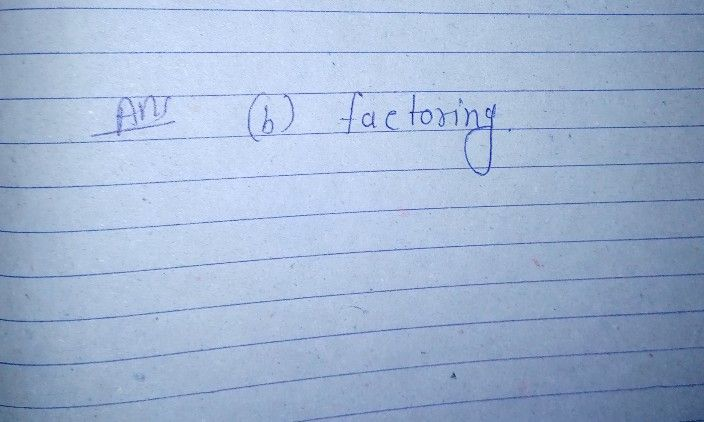Symbol
Problem$2.$ What is the most appropriate method to solve the quadratic equation $x^{2}=47$ a. extracting the square root c. completing the square b. factoring d. using the quadratic formula